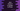# Java program to check if a given number is a prime number or not## Java program to check if a given number is a prime number or not:

In this post, we will learn how to check if a given number is a prime number or not in Java. A number is called a prime number if it is greater than 1 and is divided by 1 and the number itself.

For example, 2, 3, 5, 7, and 11 are the five starting prime numbers.

We will write a program that will take a number as the input and print if it is a prime number or not.

### Method 1: By using a loop:

We can use a loop that will run from 2 to number/2. For each value in the loop, it will check if the number is divisible by that value or not. If yes, it is not a prime number. So, it will return false.

If no number is found, it will return true i.e. it is a prime number.

Before running the loop, we will do a check for 0 and 1. Both of these are not prime numbers. So, if the parameter is 0 or 1, it will return false.

Let’s write down the program:

``````import java.util.Scanner;

class Main {
public static boolean isPrime(int n) {
if (n == 0 || n == 1) return false;

for (int i = 2; i <= n / 2; i++) {
if (n % i == 0) return false;
}
return true;
}

public static void main(String[] args) {
int n;

Scanner sc = new Scanner(System.in);
System.out.println("Enter a number: ");
n = sc.nextInt();

if (isPrime(n)) {
System.out.println(n + " is a prime number");
} else {
System.out.println(n + " is not a prime number");
}
}
}``````

Here,

• isPrime method is used to check if a number is a prime number or not. The program takes a number as an input from the user and calls isPrime to check if the number is a prime number or not.
• Based on the result of isPrime, it will print a message that the number is a prime number or it is not a prime number.

If you run this program, it will give outputs as below:

``````Enter a number:
17
17 is a prime number``````

### Method 2: Iterating to the square root of a number:

We can reduce the iteration by iterating to the square root of a number instead of half of that number. The Math class in Java provides a method called sqrt that can be used to get the square root of a number.

Let’s change the above example with Math.sqrt:

``````import java.util.Scanner;

class Main {
public static boolean isPrime(int n) {
if (n == 0 || n == 1) return false;

for (int i = 2; i <= Math.sqrt(n); i++) {
if (n % i == 0) return false;
}
return true;
}

public static void main(String[] args) {
int n;

Scanner sc = new Scanner(System.in);
System.out.println("Enter a number: ");
n = sc.nextInt();

if (isPrime(n)) {
System.out.println(n + " is a prime number");
} else {
System.out.println(n + " is not a prime number");
}
}
}``````

Here, the for loop starts from 2 and runs till Math.sqrt(number). If you run this program, it will print the below output:

``````Enter a number:
19
19 is a prime number

Enter a number:
17
17 is a prime number``````